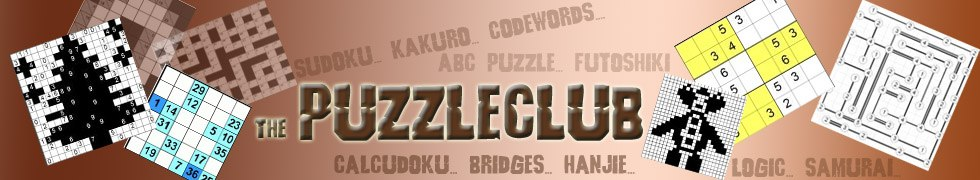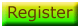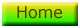# Fillomino Tips

Welcome to the Fillomino Tips section of the site, the place where we give you some hints, tips and strategies on how to solve Fillomino puzzles. We are currently adding to this section!

There are several strategies you can use to help you solve a Fillomino puzzle.

## 1 - Blocks Cannot Touch

It is essential to bear in mind the rule that blocks of the same value cannot touch when solving a puzzle, you will use this rule frequently. Look at the sample part of puzzle below, which shows the left hand edge of a puzzle: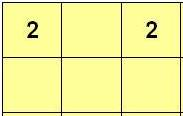Here you can see that there is a '2', then a blank, then another '2'. Because we are at the left edge of the puzzle, we know that the '2' at the top left must either move to the right or move down, to complete the block of '2'. But which way?

Well, we use the rule that no two blocks of the same value can touch to determine where it must move, downwards. If it moved to the right, we would have three '2's in a row, which would then break the rules. Thus this is the solution: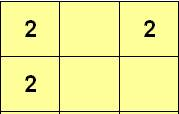## 2 - Count the Squares

It is always worth counting the total number of squares in the Fillomino puzzle, and then adding up the total of the numbers that are placed in the grid. This can help you decide whether two numbers of the same value belong to the same group or not.

Here is a very simple example, if you imagine that we had a really small puzzle that was 3 x3 in size to illustrate the point, which looked like this: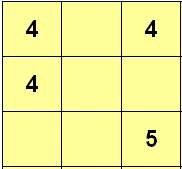In this puzzle there are only nine squares, and there are four values placed: 4,4,4 and 5. We know that two of the '4's belong to the same block of '4', as they are touching. But does the other 4? Well by counting the squares, we know the answer is 'yes'.

The '5' requires four more cells to be filled with a '5' and therefore since there are only nine squares in total, the top middle cell must also be '4' to enable a solution to be possible. Thus the solution is: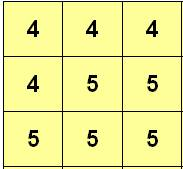## 3 - Blocked In!

It is easily overlooked that a cell is effectively trapped by other cells, and this can help you determine the cells it must fill. Look at this example from the bottom right of a puzzle: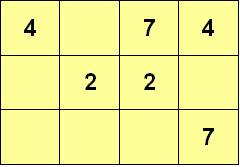Now, look at the '7' in the bottom right. We know that this must be part of a set of seven continuous cells all numbered '7'. Since it can only move in two directions, up and left, and there is only one empty cell upwards, then we know as a minimum the three cells to its left must also contain a 7. We cannot yet determine whether it fills the cell above it or not, but we know this can only be a '7' or a '1', so we mark it accordingly to work out later: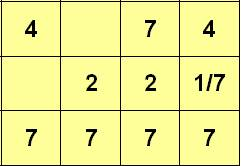# Play Fillomino Puzzles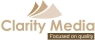©   |     |